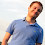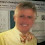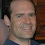## Tuesday, July 31, 2012

### A natural function with very odd properties

Last time I posted some questions. Today I post the answer that I know.

1. Is there a subset of [0,1] that is uncountable and has measure 0?  YES- take the Cantor Set.  Many readers knew this.
2. Is there such a set that is natural? Some comments thought the Cantor Set was natural. I disagree, however
this is a matter of opinion and taste.
3. Is there a function that is continuous everywhere and differential nowhere?  YES- take the Weierstrass Function.  Many readers knew this.
4. Is there such a function that is natural? Some think the Weierstrass Function is natural. I disagree because it was constructed for the sole reason to be continuous everywhere and differential nowhere.  But again, there are those who think it is natural and this is a matter of opinion.
5. Is there a function that has derivative 0 at almost every points of [0,1] even though it is strictly increasing?
YES- take Cantor Functions,
also known as the Devil's staircase. Some readers knew this.
6. Is there a natural such function? YES- and this was the motivation for the post. (I also didn't want to have the example I read about mentioned in the comments of the last post which is why I wanted all posts to be non-anonymous- so if I blocked one I could email the author WHY I blocked it.)

The function I will talk about came about because of a real problem having nothing to do with finding weird functions. Hence I think it is unambiguously natural.

I base my exposition on the exposition in
How to Gamble if you Must
by Kyle Siegrist. The original source is from the classic book Inequalities for Stochastic Processes: How to Gamble
if you must
by Dubbins and Savage. Classic or not, unless that book is free online the future
will credit Siegrist with the result (even though Siegrist references Dubbins and Savage.)

Consider the following game: Let 0 < p < 0.5.
Let 0 ≤ x ≤ 1. We will view p as a probability and x as how
You place a bet (which has to be ≤ what you have).
A coin is flipped with prob of WIN being p and of LOSS being 1-p.
If you win you double your bet. If you lose you lose your bet.
You repeat until you have 0 or you have 1.

You could play TIMIDLY: bet a fixed small amount every time.
This is analyzed. In the paper and is interesting.

You could play BOLDLY: if you have < 1/2 then bet all you have,
and if you have ≥ 1/2 then bet so that if you win you'll have 1
and can stop.

If you play BOLDLY then
what is the probability that you will end with 1?
We will fix p and let F(x) be the prob if you begin with x.
(We assume 0 ≤ x ≤ 1.)
F satisfies the following recurrence:

F(x) = pF(2x) if x ∈ [0,0.5]

F(x) = p + (1-p)F(2x-1) if x  ∈ [0.5,1].

F(0)=0, F(1)=1

This is enough to define F on all rationals between 0 and 1 (use the expansion in base 2).
Then use continuity to define F on all the rationals between 0 and 1.

The paper claims that F has derivative 0 at almost every point of [0,1]
even though its strictly increasing.
I leave this for the readers (translation into English: I don't know the proof).
However, I emailed the author who emailed me the following:

There are proofs in the book Probability and Measure by Patrick
Billingsley. In the third edition, it's Example 31.1 on page 407.  The fact
that F is continuous and strictly increasing on [0, 1] is fairly easy to
see, based on the definition of the underlying random variable.  The fact
that F has derivative 0 is not as easy to see intuitively--the proof is
more of a hard analysis type proof.

The paper assumes that money is continuous and out lives are indefinitely long.
How to Gamble if you're in a Hurry
that looks at these problems from (to quote their abstract) a purely discrete, finistic, and computational viewpoint.

1.Doesn't "strictly increasing" mean x > y -> f(x) > f(y). Cantor functions are nondecreasing.

1.Ah- good point.

That raises the odd question- is there a strictly increasing
function with dervi 0 at almost every point of [0,1],
strictly increasing, and you can PROVE this easily
(the function may be contrived).

2.Can't be true if you want continuous as well.

2.For #4, as Konstantin and I pointed out last time, there is a probabilistic example that's at least as natural as the gambling game: the Wiener process aka Brownian motion in one dimension. The gambling game is neat, and of a similar spirit. By analogy with the devil's staircase, maybe the points where the F has derivative zero (or where the player loses money?) form an uncountable measure zero set. Then we'll have random walk based examples for all three beasts.

1.Please let me agree with Sasho, for the following pragmatic reason.

A central teaching of modern quantum information science is that dynamical phenomena whose coarse-grained descriptions are naturally algebraic (e.g., projective quantum measurements) are associated to informatically equivalent fine-grained descriptions (e.g, Carmichael-style quantum trajectory unravellings) whose functional descriptors generically have the paradoxical traits that Lance's post describes.

Hence, from a purely pragmatic point-of-view, students henceforth should be taught that these paradoxical functions are "natural".

It's incredibly obvious, isn't it Mandrake?   :)

3.Isn't the Cantor set natural when seen as {0,1}^Z for example? (A very natural family of objects, used in many places.)

And isn't the boundary of a fractal a natural thing? Fractals also appear in many places, where the goal is not only to "define a funny function".

4.Answer to #6 is gamma process!

I still going with the stochastic process definition of natural. So I should mention the gamma process as what I think of as the "answer" to question 6: http://en.wikipedia.org/wiki/Gamma_process. It is a pure jump process and hence only has a countable number of jumps--this means its derivative is equal to zero almost everywhere. But, since there is a chance of jump in any arbitrarilly small time interval--it is a purely increasing process. It has lots of other nice properties--but I won't go into them now. (In general, any semi-martingale can be written as a determinsitic process plus a brownian motion process plus a gamma process. I consider semi-martingales the most natural processes we can work with, and the french school of probability proves theorems of this nature.)

1."In general, any semi-martingale can be written as a determinsitic process plus a brownian motion process plus a gamma process."

Interesting. Can you give an accessible reference for this result?

5.I agree with the previous anonymous comment: If you have ever viewed real numbers in terms of their decimal expansions I don't see how you can avoid acknowledging that the (say) decimal strings that only have 0 and 1 in their expansions is natural set.

6.1) Please elaborate- what is the 1-d Brownian Motion function?
I assume its someting like, a particle starts at 0 and
at every stage it goes left or right (how much?) and so
f(t) = prob that its at place t. But I am unclear on this so
please elaborate. I agree that something like that is clearly
natural.

2) What is the Gamma Process?

1.The gamma process is such that each increment looks like a gamma random variable. So it consists of a few jumps mixed with a more small jumps mixed with a huge number of tiny jumps, etc. One of my favorite properties of the gamma process is that it is a "perfect sun shade." Meaning if you were to sit under a gamma process you would be able to see in all directions EXCEPT straight up and down. So if the sun were perfectly over head, it wouldn't block any views at all--but it would block the sun out completely.

I've rethought my answer to #2. I'm going with it being natural also. I had forgotten that zeros of a brownian motion form a perfect set and is uncountable. So I'm happy with that being natural also.

So all three have nice probabilistic answers and so show that the properties are "generic."

2.Gasarch,

1-d brownian motion is usually defined as a probability distribution on functions f:R --> R with the following properties:

* for any reals x, y, f(x) - f(y) is distributed like a gaussian random variable with mean 0 and variance (x-y)^2
* the increments f(x_1) - f(x_2), f(x_2) - f(x_3), etc., for any x_1 < x_2 < x_3, ... are independent
* f is continuous with probability 1

A classical construction by Levy shows that such things exist. Another classical result is that with probability 1, a function sampled from this distribution is continuous and non-differentiable.

Intuitively, the random variable f is a continuous time random walk on the real line: f(t) tells us the position at time t (well, and we have negative times, but let's restrict f to [0, 1) to make this easier). One can get the Brownian motion as the limit of discrete random walks with smaller and smaller steps. I.e. think of a random walk that proceeds in time steps of length delta and at each time i*delta moves to f((i-1)*delta) + N(0, delta) where N(0, delta) is a gaussian with mean 0 and variance delta^2. This defines f at points that are integer multiples of delta. To define f everywhere, interpolate linearly. Now, as delta goes to infinity, f converges to brownian motion in distribution. This in fact happens even if the steps are not guassian but follow any distribution with finite variance. This "central limit theorem" for random walks is the Donsker invariance principle.

A great referene is the book by Moerters and Peres: http://research.microsoft.com/en-us/um/people/peres/brbook.pdf

3.A correction: I meant as delta goes to 0.

7.#2 - iirc any non-empty measure 0 subset of the interval without isolated points is Cantor (modulo an increasing continuous map of the interval). Being essentially unique makes it natural, I'd say.